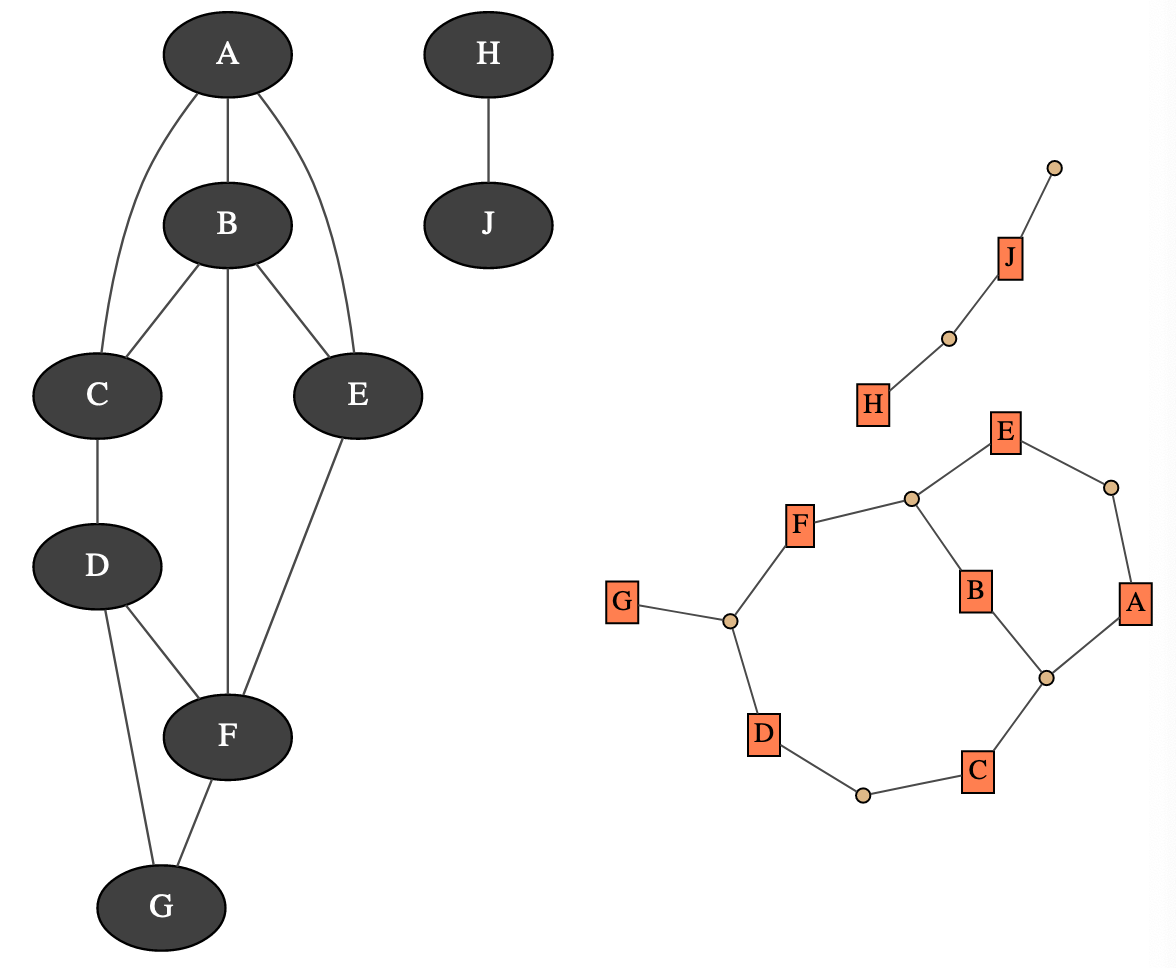# Markov Network¶A Markov network is a undirected probabilistic graphical model. It represents a joint distribution over a set of random variables. In pyAgrum, the variables are (for now) only discrete.

A Markov network uses a undirected graph to represent conditional indepencies in the joint distribution. These conditional indepencies allow to factorize the joint distribution, thereby allowing to compactly represent very large ones. Moreover, inference algorithms can also use this graph to speed up the computations.

Tutorial

Reference